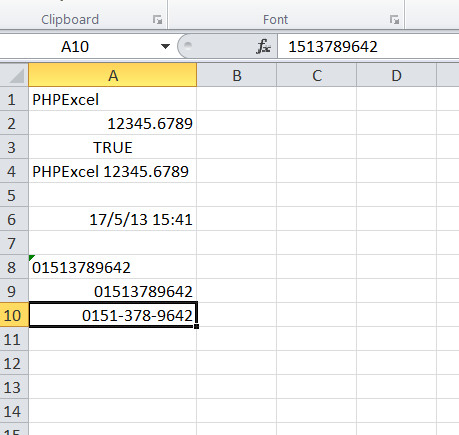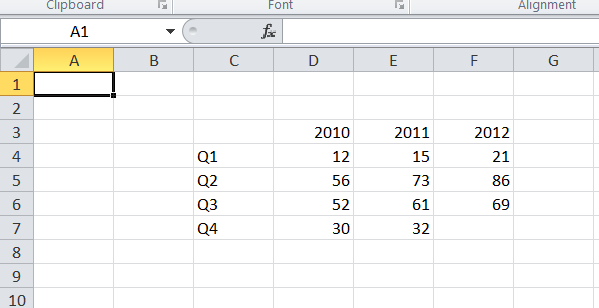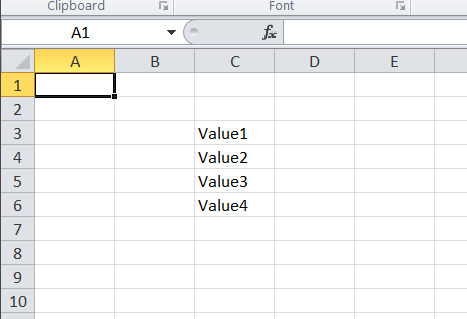# Accessing cells

Accessing cells in a Spreadsheet should be pretty straightforward. This topic lists some of the options to access a cell.

## Setting a cell value by coordinate

Setting a cell value by coordinate can be done using the worksheet's `setCellValue()` method.

``````// Set cell A1 with a string value

// Set cell A2 with a numeric value

// Set cell A3 with a boolean value

// Set cell A4 with a formula
'A4',
'=IF(A3, CONCATENATE(A1, " ", A2), CONCATENATE(A2, " ", A1))'
);
``````

Alternatively, you can retrieve the cell object, and then call the cell’s `setValue()` method:

``````\$spreadsheet->getActiveSheet()
->getCell('B8')
->setValue('Some value');
``````

### Creating a new Cell

If you make a call to `getCell()`, and the cell doesn't already exist, then PhpSpreadsheet will (by default) create the cell for you. If you don't want to create a new cell, then you can pass a second argument of false, and then `getCell()` will return a null if the cell doesn't exist.

### BEWARE: Cells assigned to variables as a Detached Reference

As an "in-memory" model, PHPSpreadsheet can be very demanding of memory, particularly when working with large spreadsheets. One technique used to reduce this memory overhead is cell caching, so cells are actually maintained in a collection that may or may not be held in memory while you are working with the spreadsheet. Because of this, a call to `getCell()` (or any similar method) returns the cell data, and a pointer to the collection. While this is not normally an issue, it can become significant if you assign the result of a call to `getCell()` to a variable. Any subsequent calls to retrieve other cells will unset that pointer, although the cell object will still retain its data values.

What does this mean? Consider the following code:

``````\$spreadSheet = new Spreadsheet();

// Set details for the formula that we want to evaluate, together with any data on which it depends
\$workSheet->fromArray(
[1, 2, 3],
null,
'A1'
);

\$cellC1 = \$workSheet->getCell('C1');
echo 'Value: ', \$cellC1->getValue(), '; Address: ', \$cellC1->getCoordinate(), PHP_EOL;

\$cellA1 = \$workSheet->getCell('A1');
echo 'Value: ', \$cellA1->getValue(), '; Address: ', \$cellA1->getCoordinate(), PHP_EOL;

echo 'Value: ', \$cellC1->getValue(), '; Address: ', \$cellC1->getCoordinate(), PHP_EOL;
``````

The call to `getCell('C1')` returns the cell at `C1` containing its value (`3`), together with its link to the collection (used to identify its address/coordinate `C1`). The subsequent call to access cell `A1` modifies the value of `\$cellC1`, detaching its link to the collection.

So when we try to display the value and address a second time, we can display its value, but trying to display its address/coordinate will throw an exception because that link has been set to null.

Note: There are some internal methods that will fetch other cells from the collection, and this too will detach the link to the collection from any cell that you might have assigned to a variable.

## Excel DataTypes

MS Excel supports 7 basic datatypes:

• string
• number
• boolean
• null
• formula
• error
• Inline (or rich text) string

By default, when you call the worksheet's `setCellValue()` method or the cell's `setValue()` method, PhpSpreadsheet will use the appropriate datatype for PHP nulls, booleans, floats or integers; or cast any string data value that you pass to the method into the most appropriate datatype, so numeric strings will be cast to numbers, while string values beginning with `=` will be converted to a formula. Strings that aren't numeric, or that don't begin with a leading `=` will be treated as genuine string values.

This "conversion" is handled by a cell "value binder", and you can write custom "value binders" to change the behaviour of these "conversions". The standard PhpSpreadsheet package also provides an "advanced value binder" that handles a number of more complex conversions, such as converting strings with a fractional format like "3/4" to a number value (0.75 in this case) and setting an appropriate "fraction" number format mask. Similarly, strings like "5%" will be converted to a value of 0.05, and a percentage number format mask applied, and strings containing values that look like dates will be converted to Excel serialized datetimestamp values, and a corresponding mask applied. This is particularly useful when loading data from csv files, or setting cell values from a database.

Formats handled by the advanced value binder include:

• TRUE or FALSE (dependent on locale settings) are converted to booleans.
• Numeric strings identified as scientific (exponential) format are converted to numbers.
• Fractions and vulgar fractions are converted to numbers, and an appropriate number format mask applied.
• Percentages are converted to numbers, divided by 100, and an appropriate number format mask applied.
• Dates and times are converted to Excel timestamp values (numbers), and an appropriate number format mask applied.
• When strings contain a newline character (`\n`), then the cell styling is set to wrap.

You can read more about value binders later in this section of the documentation.

### Setting a formula in a Cell

As stated above, if you store a string value with the first character an `=` in a cell. PHPSpreadsheet will treat that value as a formula, and then you can evaluate that formula by calling `getCalculatedValue()` against the cell.

There may be times though, when you wish to store a value beginning with `=` as a string, and that you don't want PHPSpreadsheet to evaluate as though it was a formula.

To do this, you need to "escape" the value by setting it as "quoted text".

``````// Set cell A4 with a formula
'A4',
'=IF(A3, CONCATENATE(A1, " ", A2), CONCATENATE(A2, " ", A1))'
);
->getStyle()->setQuotePrefix(true);
``````

Then, even if you ask PHPSpreadsheet to return the calculated value for cell `A4`, it will return `=IF(A3, CONCATENATE(A1, " ", A2), CONCATENATE(A2, " ", A1))` as a string, and not try to evaluate the formula.

### Setting a date and/or time value in a cell

Date or time values are held as timestamp in Excel (a simple floating point value), and a number format mask is used to show how that value should be formatted; so if we want to store a date in a cell, we need to calculate the correct Excel timestamp, and set a number format mask.

``````// Get the current date/time and convert to an Excel date/time
\$dateTimeNow = time();
// Set cell A6 with the Excel date/time value
'A6',
\$excelDateValue
);
// Set the number format mask so that the excel timestamp will be displayed as a human-readable date/time
->getNumberFormat()
->setFormatCode(
);
``````

### Setting a number with leading zeroes

By default, PhpSpreadsheet will automatically detect the value type and set it to the appropriate Excel numeric datatype. This type conversion is handled by a value binder, as described in the section of this document entitled "Using value binders to facilitate data entry".

Numbers don't have leading zeroes, so if you try to set a numeric value that does have leading zeroes (such as a telephone number) then these will be normally be lost as the value is cast to a number, so "01513789642" will be displayed as 1513789642.

There are two ways you can force PhpSpreadsheet to override this behaviour.

Firstly, you can set the datatype explicitly as a string so that it is not converted to a number.

``````// Set cell A8 with a numeric value, but tell PhpSpreadsheet it should be treated as a string
'A8',
"01513789642",
);
``````

Alternatively, you can use a number format mask to display the value with leading zeroes.

``````// Set cell A9 with a numeric value
// Set a number format mask to display the value as 11 digits with leading zeroes
->getNumberFormat()
->setFormatCode(
'00000000000'
);
``````

With number format masking, you can even break up the digits into groups to make the value more easily readable.

``````// Set cell A10 with a numeric value
// Set a number format mask to display the value as 11 digits with leading zeroes
->getNumberFormat()
->setFormatCode(
'0000-000-0000'
);
``````Note: that not all complex format masks such as this one will work when retrieving a formatted value to display "on screen", or for certain writers such as HTML or PDF, but it will work with the true spreadsheet writers (Xlsx and Xls).

## Setting a range of cells from an array

It is also possible to set a range of cell values in a single call by passing an array of values to the `fromArray()` method.

``````\$arrayData = [
[NULL, 2010, 2011, 2012],
['Q1',   12,   15,   21],
['Q2',   56,   73,   86],
['Q3',   52,   61,   69],
['Q4',   30,   32,    0],
];
->fromArray(
\$arrayData,  // The data to set
NULL,        // Array values with this value will not be set
'C3'         // Top left coordinate of the worksheet range where
//    we want to set these values (default is A1)
);
``````If you pass a 2-d array, then this will be treated as a series of rows and columns. A 1-d array will be treated as a single row, which is particularly useful if you're fetching an array of data from a database.

``````\$rowArray = ['Value1', 'Value2', 'Value3', 'Value4'];
->fromArray(
\$rowArray,   // The data to set
NULL,        // Array values with this value will not be set
'C3'         // Top left coordinate of the worksheet range where
//    we want to set these values (default is A1)
);
``````If you have a simple 1-d array, and want to write it as a column, then the following will convert it into an appropriately structured 2-d array that can be fed to the `fromArray()` method:

``````\$rowArray = ['Value1', 'Value2', 'Value3', 'Value4'];
\$columnArray = array_chunk(\$rowArray, 1);
->fromArray(
\$columnArray,   // The data to set
NULL,           // Array values with this value will not be set
'C3'            // Top left coordinate of the worksheet range where
//    we want to set these values (default is A1)
);
``````## Retrieving a cell value by coordinate

To retrieve the value of a cell, the cell should first be retrieved from the worksheet using the `getCell()` method. A cell's value can be read using the `getValue()` method.

``````// Get the value from cell A1
``````

This will retrieve the raw, unformatted value contained in the cell.

If a cell contains a formula, and you need to retrieve the calculated value rather than the formula itself, then use the cell's `getCalculatedValue()` method. This is further explained in the calculation engine.

``````// Get the value from cell A4
``````

Alternatively, if you want to see the value with any cell formatting applied (e.g. for a human-readable date or time value), then you can use the cell's `getFormattedValue()` method.

``````// Get the value from cell A6
``````

## Setting a cell value by column and row

Setting a cell value by coordinate can be done using the worksheet's `setCellValueByColumnAndRow()` method.

``````// Set cell A5 with a string value
``````

Note: that column references start with `1` for column `A`.

## Retrieving a cell value by column and row

To retrieve the value of a cell, the cell should first be retrieved from the worksheet using the `getCellByColumnAndRow()` method. A cell’s value can be read again using the following line of code:

``````// Get the value from cell B5
``````

If you need the calculated value of a cell, use the following code. This is further explained in the calculation engine.

``````// Get the value from cell A4
``````

## Retrieving a range of cell values to an array

It is also possible to retrieve a range of cell values to an array in a single call using the `toArray()`, `rangeToArray()` or `namedRangeToArray()` methods.

``````\$dataArray = \$spreadsheet->getActiveSheet()
->rangeToArray(
'C3:E5',     // The worksheet range that we want to retrieve
NULL,        // Value that should be returned for empty cells
TRUE,        // Should formulas be calculated (the equivalent of getCalculatedValue() for each cell)
TRUE,        // Should values be formatted (the equivalent of getFormattedValue() for each cell)
TRUE         // Should the array be indexed by cell row and cell column
);
``````

These methods will all return a 2-d array of rows and columns. The `toArray()` method will return the whole worksheet; `rangeToArray()` will return a specified range or cells; while `namedRangeToArray()` will return the cells within a defined `named range`.

## Looping through cells

### Looping through cells using iterators

The easiest way to loop cells is by using iterators. Using iterators, one can use foreach to loop worksheets, rows within a worksheet, and cells within a row.

Below is an example where we read all the values in a worksheet and display them in a table.

``````\$reader = \PhpOffice\PhpSpreadsheet\IOFactory::createReader('Xlsx');

echo '<table>' . PHP_EOL;
foreach (\$worksheet->getRowIterator() as \$row) {
echo '<tr>' . PHP_EOL;
\$cellIterator = \$row->getCellIterator();
\$cellIterator->setIterateOnlyExistingCells(FALSE); // This loops through all cells,
//    even if a cell value is not set.
// By default, only cells that have a value
//    set will be iterated.
foreach (\$cellIterator as \$cell) {
echo '<td>' .
\$cell->getValue() .
'</td>' . PHP_EOL;
}
echo '</tr>' . PHP_EOL;
}
echo '</table>' . PHP_EOL;
``````

Note that we have set the cell iterator's `setIterateOnlyExistingCells()` to FALSE. This makes the iterator loop all cells within the worksheet range, even if they have not been set.

The cell iterator will return a `null` as the cell value if it is not set in the worksheet. Setting the cell iterator's `setIterateOnlyExistingCells()` to `false` will loop all cells in the worksheet that can be available at that moment. This will create new cells if required and increase memory usage! Only use it if it is intended to loop all cells that are possibly available.

### Looping through cells using indexes

One can use the possibility to access cell values by column and row index like `[1, 1]` instead of `'A1'` for reading and writing cell values in loops.

Note: In PhpSpreadsheet column index and row index are 1-based. That means `'A1'` ~ `[1, 1]`

Below is an example where we read all the values in a worksheet and display them in a table.

``````\$reader = \PhpOffice\PhpSpreadsheet\IOFactory::createReader('Xlsx');

// Get the highest row and column numbers referenced in the worksheet
\$highestRow = \$worksheet->getHighestRow(); // e.g. 10
\$highestColumn = \$worksheet->getHighestColumn(); // e.g 'F'
\$highestColumnIndex = \PhpOffice\PhpSpreadsheet\Cell\Coordinate::columnIndexFromString(\$highestColumn); // e.g. 5

echo '<table>' . "\n";
for (\$row = 1; \$row <= \$highestRow; ++\$row) {
echo '<tr>' . PHP_EOL;
for (\$col = 1; \$col <= \$highestColumnIndex; ++\$col) {
\$value = \$worksheet->getCellByColumnAndRow(\$col, \$row)->getValue();
echo '<td>' . \$value . '</td>' . PHP_EOL;
}
echo '</tr>' . PHP_EOL;
}
echo '</table>' . PHP_EOL;
``````

Alternatively, you can take advantage of PHP's "Perl-style" character incrementors to loop through the cells by coordinate:

``````\$reader = \PhpOffice\PhpSpreadsheet\IOFactory::createReader('Xlsx');

// Get the highest row number and column letter referenced in the worksheet
\$highestRow = \$worksheet->getHighestRow(); // e.g. 10
\$highestColumn = \$worksheet->getHighestColumn(); // e.g 'F'
// Increment the highest column letter
\$highestColumn++;

echo '<table>' . "\n";
for (\$row = 1; \$row <= \$highestRow; ++\$row) {
echo '<tr>' . PHP_EOL;
for (\$col = 'A'; \$col != \$highestColumn; ++\$col) {
echo '<td>' .
\$worksheet->getCell(\$col . \$row)
->getValue() .
'</td>' . PHP_EOL;
}
echo '</tr>' . PHP_EOL;
}
echo '</table>' . PHP_EOL;
``````

Note that we can't use a `<=` comparison here, because `'AA'` would match as `<= 'B'`, so we increment the highest column letter and then loop while `\$col !=` the incremented highest column.

## Using value binders to facilitate data entry

Internally, PhpSpreadsheet uses a default `\PhpOffice\PhpSpreadsheet\Cell\IValueBinder` implementation (\PhpOffice\PhpSpreadsheet\Cell\DefaultValueBinder) to determine data types of entered data using a cell's `setValue()` method (the `setValueExplicit()` method bypasses this check).

Optionally, the default behaviour of PhpSpreadsheet can be modified, allowing easier data entry. For example, a `\PhpOffice\PhpSpreadsheet\Cell\AdvancedValueBinder` class is available. It automatically converts percentages, number in scientific format, and dates entered as strings to the correct format, also setting the cell's style information. The following example demonstrates how to set the value binder in PhpSpreadsheet:

``````/** PhpSpreadsheet */
require_once 'src/Boostrap.php';

// Set value binder

Creating your own value binder is easy. When advanced value binding is required, you can implement the `\PhpOffice\PhpSpreadsheet\Cell\IValueBinder` interface or extend the `\PhpOffice\PhpSpreadsheet\Cell\DefaultValueBinder` or `\PhpOffice\PhpSpreadsheet\Cell\AdvancedValueBinder` classes.